#Introduction and MotivationThe differential equations are very much helpful in many areas of science. But most of interesting real life problems involve more than one unknown function. Therefore, the use of system of differential equations is very useful. Without loss of generality, we will concentrate on systems of two differential equationsAs a motivation let us consider an island with two type of species: Rabbits and Fox. Clearly one plays the role of predator while the other one the role of a prey. If we are interested to model the populations growths of both species, then we have to keep in mind that if, for example, the population of the Fox increases, then the Rabbit population will be affected. So the rate of change of the population of one type will depend on the actual population of the other type. For example, in the absence of the Rabbit population, the Fox population will decrease (and fast) to face a certain extinction. Something that most of us would like to avoid. A model for this Predator-Prey problem was developed by Lotka (in 1925) and Volterra (in 1926) and is known as the Lotka-Volterra systemwhere R(t) measures the Rabbit population, F(t) measures the Fox population, and all the involved constant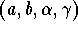are positive numbers. Note that a and b are the growth rate of the prey, and the death rate of the predator.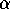and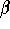are measures of the effect of the interaction between the Rabbits and The Fox.

Note that in the Lotka-Volterra system, the variable t is missing. This kind of system is called autonomous system and are writtenPhase Plane

Let us go back to the general caseA solution to this system is the couple of functions (x(t),y(t)) which satisfy both differential equations of the system. When we change the variable t, then we get a set of points on the xy-plane which, in physics, we usually call a trajectory. The moving object has the coordinates (x(t),y(t)) at time t. The velocity to the trajectory at time t is given by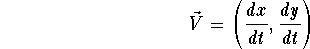Note that we do not need to know the solution (x(t),y(t) to determine the velocity vector at time t. Indeed, we have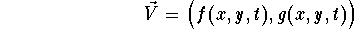as long as we know x, y, and t. In particular, we can draw all the velocity vectors everywhere on the plane for the autonomous systems. This is known as the vector field.
The vector field should be understood as the analogue of the direction field for differential equations.

Example. Draw the vector field for the predator-prey problemFor example, for the point (x,y)= (2,1), we have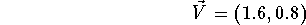If we put more velocity vectors, we get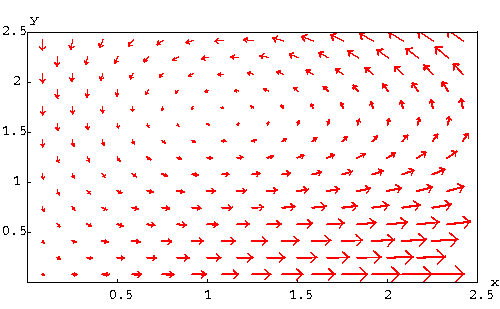It is clear that the length of the velocity vectors may affect our understanding of the solutions. If we are only interested by the direction of the motion not its speed, then it is natural to fix a length for these vectors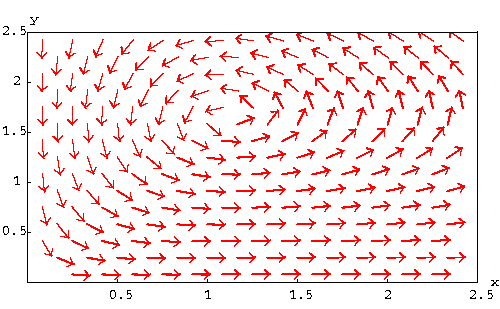Compare the two pictures.

Remark. Note that for the predator-prey problem, we may be interested to find out whether one of the two species is facing extinction or not. In other words, we may be interested to study the functions x(t) and y(t) separately. In other words, two more graphs are naturally associated to a system. For the above example, the graph of the solution which satisfies the condition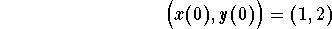is drawn below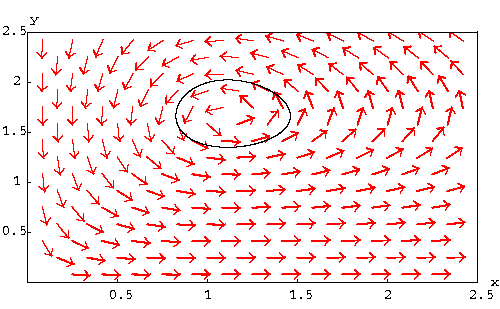and the graphs of x(t) and y(t) are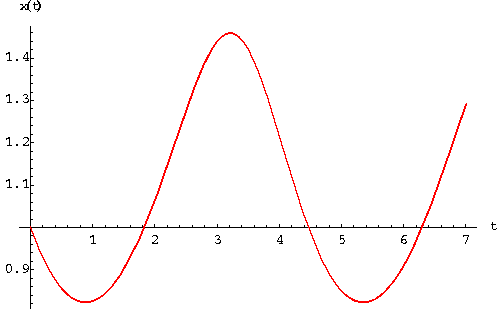and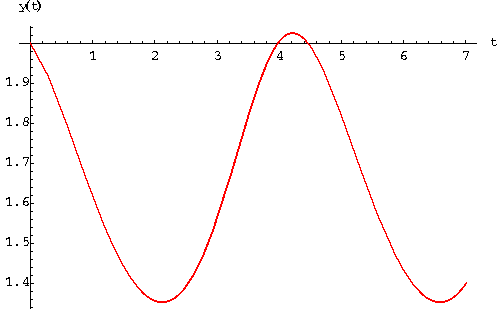Equilibrium Solutions.
An equilibrium solution (or critical solution) of an autonomous system is the trajectory of a moving-object with no motion, that is the object is not moving. In this case, the velocity vector is basically equal to 0. Consider the autonomous systemThe equilibrium solutions are the algebraic solutions of the system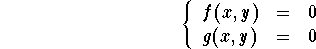The equilibrium solutions are also called equilibrium points since the associated trajectories are exactly points on the phase plane.

Example. Find the equilibrium points of the Duffin system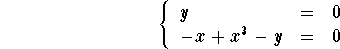Plugging y=0 in the second equation, we get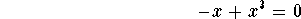which gives x = 0, or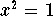. Hence the equilibrium points are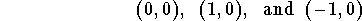### If you would like more practice, click on Example.[Differential Equations] [First Order D.E.]
[Geometry] [Algebra] [Trigonometry ]
[Calculus] [Complex Variables] [Matrix Algebra]S.O.S MATHematics home page

Do you need more help? Please post your question on our S.O.S. Mathematics CyberBoard.Author: Mohamed Amine Khamsi